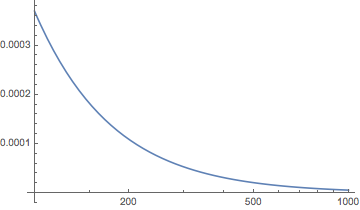Finding a tight upper bound of $\int_0^\infty e^{-x^2/2-a(1-e^{-x})}dx,\ a>0$, as a function of $a$

Is there a method to find a tight upper bound on the given integral? Note that the integral is upper bounded by $\sqrt{\pi/2}$, and thus converges. I first thought about applying Laplace's method. However, the function $-x^2/2-a(1-e^{-x})$ is decreasing and achieves maximum at $x=0$ which is an endpoint of the domain of integration. As a result, I don't think using Laplace method is a good idea to find an upper bound. Numerical evaluation indicates an asymptotic of $\sim \frac{1}{a}$, but I am not sure how to proceed to say anything about an upper bound in terms of $a$. Any ideas? Thanks in advance.

The Laplace method is applicable to the integral $F(\lambda):=\int\limits_a^b f(x)e^{\lambda S(x)}$ in the case $\max\limits_{x\in [a,b]}S(x)=S(a)$ too. For example, this is described in V. Zorich, Mathematical Analysis II Chap. XIX, Par. 2.4, Theorem 1.

For the user's convenience here is a part of its statement

Then $$F(\lambda)=\frac {f(a)} {-S'(a)} \frac {e^{\lambda S(a)}} \lambda (1+O(\lambda^{-1})),\, \lambda \to \infty.$$

In the case under consideration $f(x):=e^{-x^2/2},\,S(x):=e^{-x}-1,\,\lambda:=a.$ This gives the asymptotics $\frac 1 a \left(1+O( \frac 1 a) \right) ,\, a\to \infty.$

• Higher order terms can easily be found using the expansion DLMF $I(a)\sim a^{-1}+a^{-2}+a^{-3}$ with an error less than $3.10^{-4}$ for $a>10$. Feb 2 '18 at 14:19
• @user64494 Do you have any advice on what to do when I have a function such that $S'(a)=\infty$, for example, if $a=0$, $S(x)=-\sqrt{x}$. Though this case can be handled differently, I just want to see if the Laplace method can be applied here in any way. Feb 3 '18 at 10:48
• @Samrat Mukhopadhyay: Can you present the whole integral you ask about? Did you try the change $t=\sqrt{x}?$ Feb 3 '18 at 10:55
• Actually, the integral I was asking about in the previous comment is $\int_0^\infty e^{-a\sqrt{x}-x^2/2} dx$. Although it can be easily upper bounded as $\int_0^\infty e^{-a\sqrt{x}}dx$, which then is just $2/\lambda^2$, I want to see if Laplace's method can be applied to this case in any way so that I get a feel about applying this method to more general functions. Feb 3 '18 at 10:59
• @Samrat Mukhopadhyay: I don't know whether the Laplace's method directly works for $S(x):=-\sqrt{x}$. There are some generalisations of that method for $\int_a^b e^{S(\lambda,x)}\,dx$. Feb 3 '18 at 11:26

The integral $$I(a)=\int_0^\infty e^{-x^2/2-a(1-e^{-x})}dx$$ approaches $1/a$ from above, for an upper bound I tried $$B(a)=a^{1/a-1}$$ which seems to work, see this plot of $B(a)-I(a)$ versus $a$:• Can you kindly elaborate how you obtained the bounds? Feb 3 '18 at 5:19